# Commutative Property of Multiplication

The commutative property of multiplication states that you can multiply numbers in any order.

In English to commute means to travel or to change location.

In math, the commutative property of multiplication allows us to change the places of factors in a product.

For example:

2 x 3 = 6
3 x 2 = 6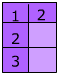and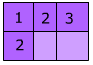This means that 2 x 3 = 3 x 2

The product is the same, only the factors have changed places.

Example : Is 6 x 7 = 7 x 6 a true statement?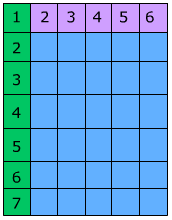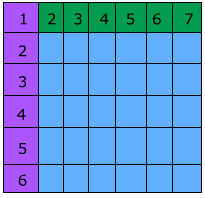Answer: Yes, because you can multiply the numbers in either order.

Example: Is 5 x 8 = 8 x 5 a true statement?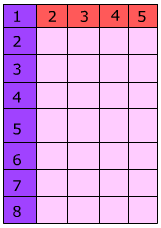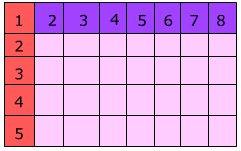Answer: Yes, because you can multiply the numbers in any order. Example: Use commutative property of multiplication to rewrite 5 x 4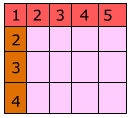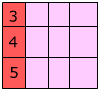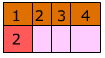5 x 4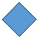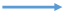Answer: 4 x 5

Example: Use commutative property of multiplication to rewrite 6 x 3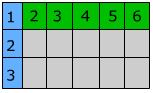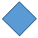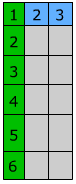6 x 3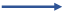Answer: 3 x 6

Example: What is the missing number in 9 x 4 = 4 x ____?

Because with the commutative property of multiplication we can exchange the numbers and

9 * 4 = 4 * 9

Example: What is the missing number in 7 x 3 = 3 x ___ ?

Because we can multiply the factors in either order, and 7 x 3 = 3 x 7

Now that you know that the numbers can be multiplied in any order, you can change the factors to multiply in the order that you want.

Math
algebra
Distributive Property
Inverse Properties of Addition and Multiplication

To link to this Commutative Property of Multiplication page, copy the following code to your site: